# HABS: Game-by-game, Player-by-player Ratings and Ratios

By Kicks @Chrisboucher73

Covering the Canadiens last 17 games
The graphs included here represent each player's risk/reward rating and ratio from each of the game's indicated. A green bar indicates a game the Habs won, while a red bar shows a loss.
Risk/reward rating is calculated by subtracting the amount of failed plays a player contributes per-minute played from the number of successful plays per-minute played. The higher the number, the better a player has performed. Because ice-time is used in this calculation, the level of involvement a player contributes will impact his rating. The more involved a player is (events per-minute) the higher he can push his risk/reward rating. A negative rating indicates that a player has produced more unsuccessful plays than successful plays.
Ratio is calculated by dividing the number of failed plays a player contributes from the number of successful plays. The higher the number, the better a player has performed. Ice-time is not included in this calculation. A ratio below 1.00 indicates that a player has produced more unsuccessful plays than successful plays.
HABS GAME-BY-GAME
The Montreal Canadiens average risk/reward rating prior to the Olympics was 1.69; since the games it's been 1.75. The Habs winning percentage prior to the Olympics was .542 ; in the games included here it is .588.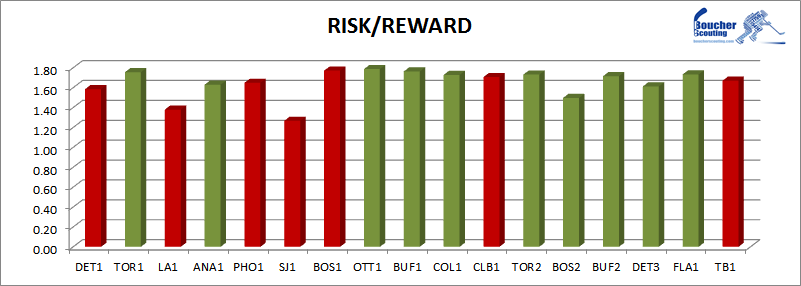The Canadiens average ratio prior to the Olympics was 2.29; since the games it's been 2.52. Again, the Habs winning percentage prior to the Olympics was .542 ; in the games included here it is .588.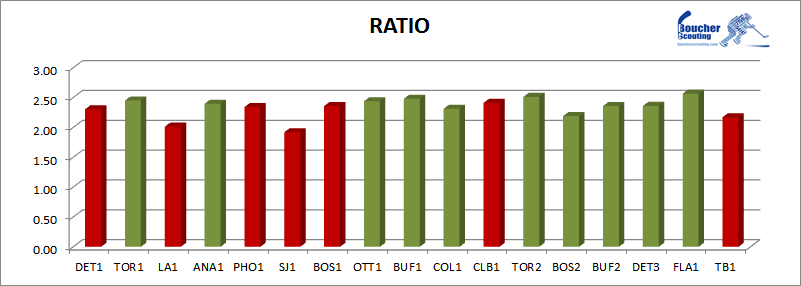DOUGLAS MURRAY
The average risk/reward rating for Habs DEFENSEMEN prior to the Olympics was 2.11; since the games it's been 2.00. Murray's average ratio rating prior to the Olympics was 1.65; since the games it's been 1.48.
SInce the Olympics, Murray's rating  has been above the average for his position only once.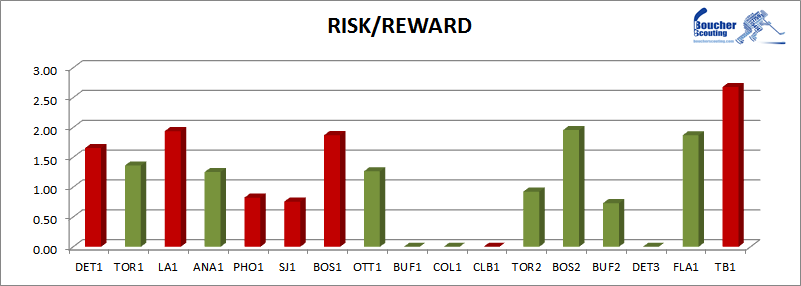The average risk/reward rating for Habs DEFENSEMEN prior to the Olympics was 2.84; since the games it's been 2.76.  Murray's average ratio prior to the Olympics was 2.36; since the games it's been 2.14.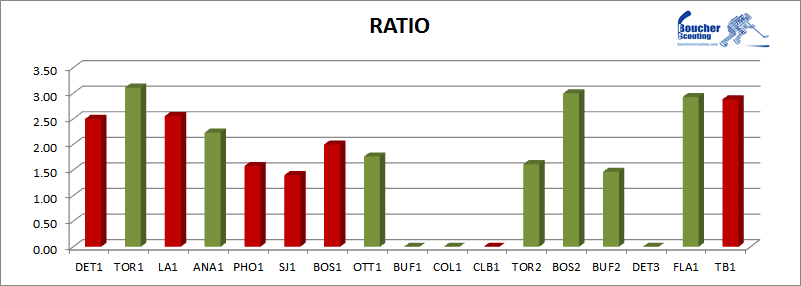BRANDON PRUST
The average risk/reward rating for Habs WINGERS  prior to the Olympics was 1.26; since the games it's been 1.38. Prust's average risk/reward rating prior to the Olympics was 1.35; since the games it's been 2.02.
Prust's rating was above the average for his position during 3 of the 4 games he's played.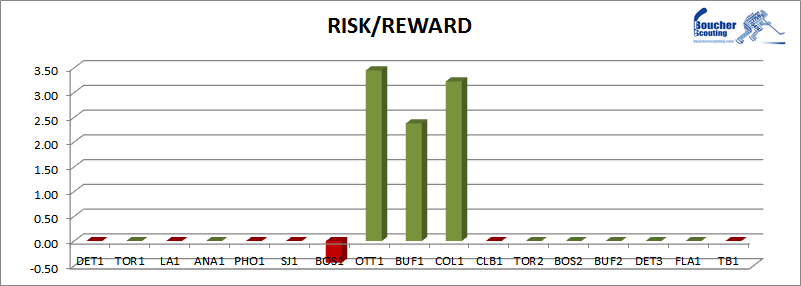The average ratio for Habs WINGERS prior to the Olympics was 1.88; since the games it's been 2.02.  Prust's average ratio prior to the Olympics was 2.00; since the games it's been 2.78.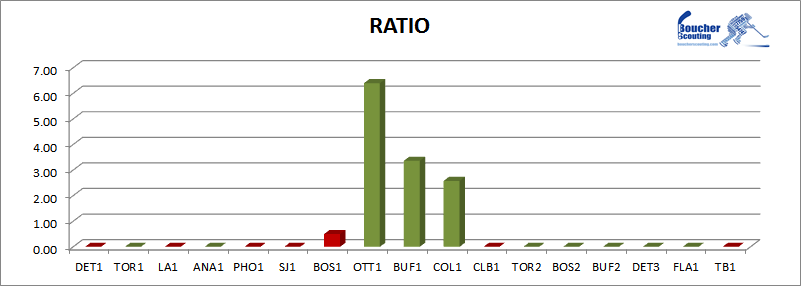GALLAGHER RISK/REWARD
The average risk/reward rating for Habs WINGERS  prior to the Olympics was 1.26; since the games it's been 1.38. Gallagher's average risk/reward rating prior to the Olympics was 1.42; since the games it's been 1.72.
Since the Olympics, Gallagher's rating has been above the average for his position 12 times; but only twice in the last 7 games.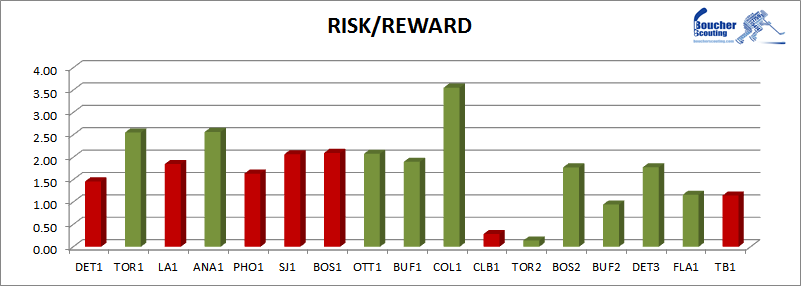The average ratio for Habs WINGERS prior to the Olympics was 1.88; since the games it's been 2.02.  Gallagher's average ratio prior to the Olympics was 1.92; since the games it's been 2.23.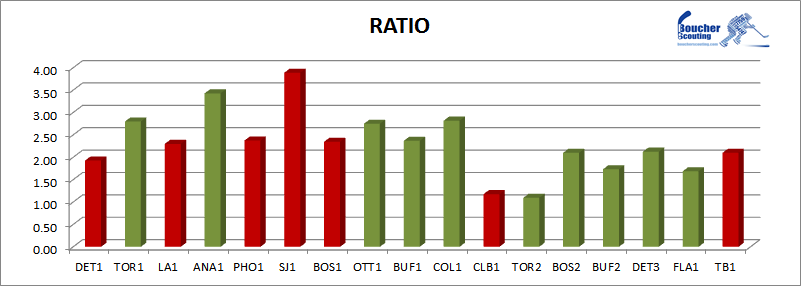PLEKANEC RISK/REWARD
The average risk/reward rating for Habs CENTRES  prior to the Olympics was 1.57; since the games it's been 1.52. Plekanec's average risk/reward rating prior to the Olympics was 1.48; since the games it's been 1.54.
Since the Olympics, Plekanec's rating has been above the average for his position in 8 of 16 games.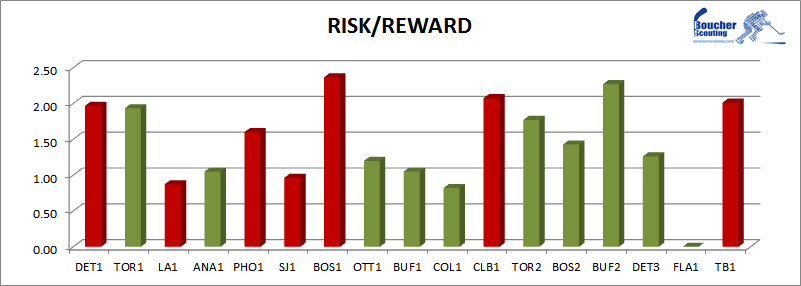The average ratio for Habs CENTRES prior to the Olympics was 2.20; since the games it's been 2.22.  Plekanec's average ratio prior to the Olympics was 2.12; since the games it's been 2.35.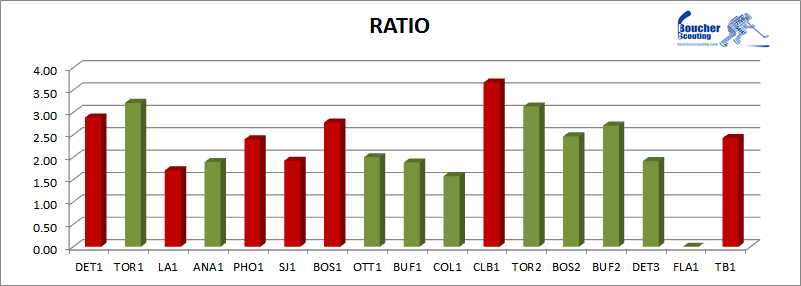GEORGE PARROS
The average risk/reward rating for Habs WINGERS  prior to the Olympics was 1.26; since the games it's been 1.38. Parros' average risk/reward rating prior to the Olympics was 0.62; since the games it's been 1.14.
Since the games, Parros' rating has never moved above the average for his position.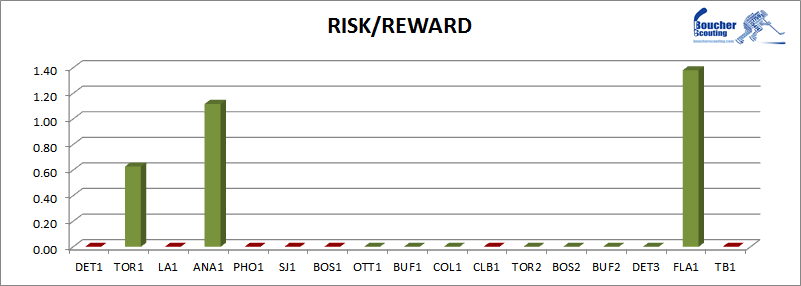The average ratio for Habs WINGERS prior to the Olympics was 1.88; since the games it's been 2.02.  Parros' average ratio prior to the Olympics was 1.48; since the games it's been 1.89.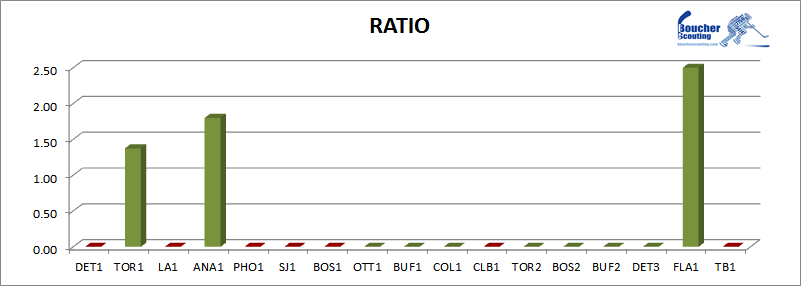RENE BOURQUE
The average risk/reward rating for Habs WINGERS  prior to the Olympics was 1.26; since the games it's been 1.38. Parros' average risk/reward rating prior to the Olympics was 0.85; since the games it's been 0.69.
Since the Olympics, Bourque's rating has been above the average for his position only twice.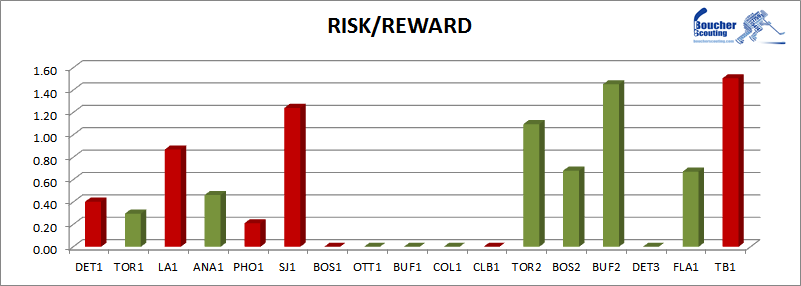The average ratio for Habs WINGERS prior to the Olympics was 1.88; since the games it's been 2.02.  Bourque's average ratio prior to the Olympics was 1.61; since the games it's been 1.46.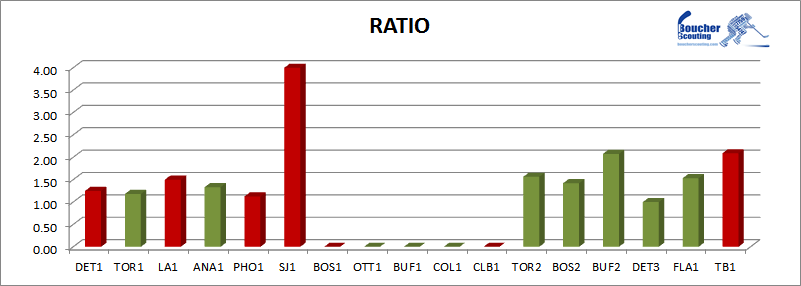THOMAS VANEK
The average risk/reward rating for Habs WINGERS  prior to the Olympics was 1.26; since the games it's been 1.38. Vanek's risk/reward rating since his arriveal has been 1.16.
Since his arrival, Vanek's rating has been above the average for his position 5 times. Four of those instances have occurred in the last 6 games.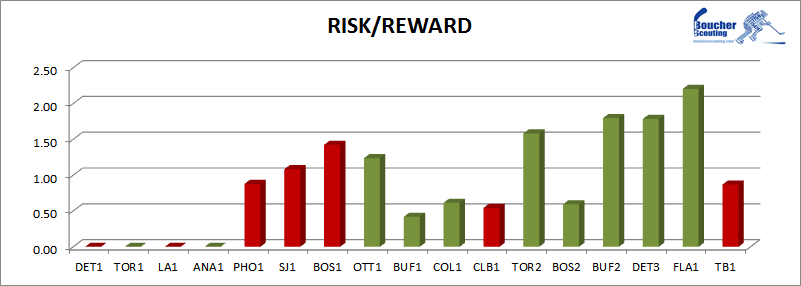The average ratio for Habs WINGERS prior to the Olympics was 1.88; since the games it's been 2.02.  Vanek's ratio since his arrival has been 1.82.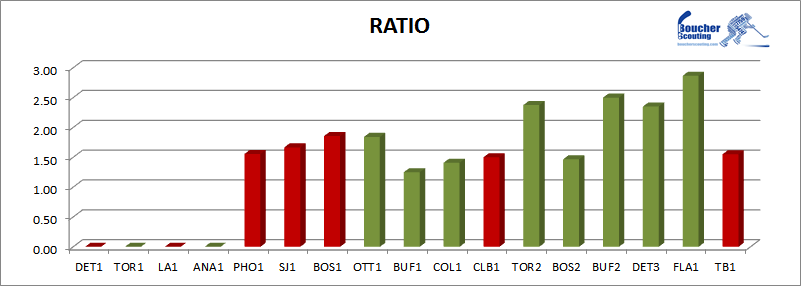BRIAN GIONTA
The average risk/reward rating for Habs WINGERS  prior to the Olympics was 1.26; since the games it's been 1.38. Gionta's average risk/reward rating prior to the Olympics was 1.26; since the games it's been 1.35.
Since the Olympics, Gionta's rating has been above the average among wingers 6 times. Four of those instances have occurred over the last 7 games.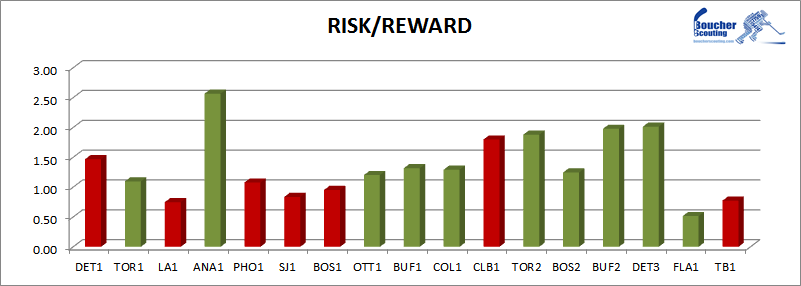The average ratio for Habs WINGERS prior to the Olympics was 1.88; since the games it's been 2.02.  Gionta's average ratio prior to the Olympics was 1.93; since the games it's been 2.04.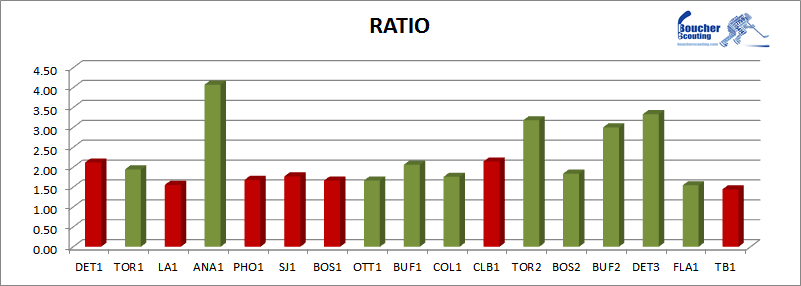DALE WEISE
The average risk/reward rating for Habs WINGERS  prior to the Olympics was 1.26; since the games it's been 1.38. Weise's average risk/reward rating prior to the Olympics was 1.30; since the games it's been 1.60. Since the games, Weise's rating has been above the average for his position during 4 of 10 games.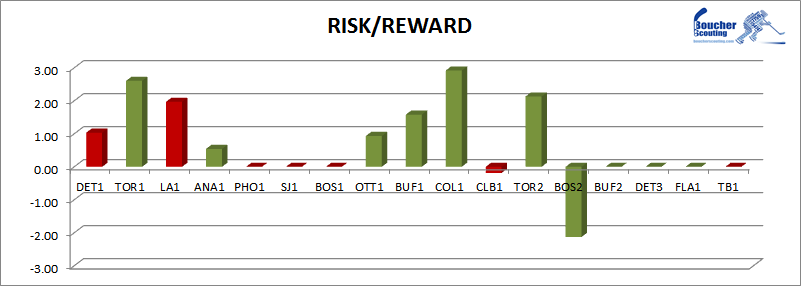The average ratio for Habs WINGERS prior to the Olympics was 1.88; since the games it's been 2.02.  Weise's average ratio prior to the Olympics was 1.92; since the games it's been 2.52.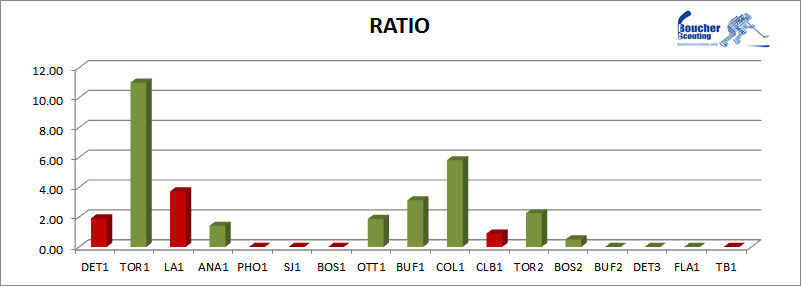JARRED TINORDI
The average risk/reward rating for Habs DEFENSEMEN prior to the Olympics was 2.11; since the games it's been 2.00. Tinordi's average risk/reward rating prior to the Olympics was 1.33; since the games it's been 1.69.
Since the Olympics, Tinordi's rating has been above average during 5 of 12 games.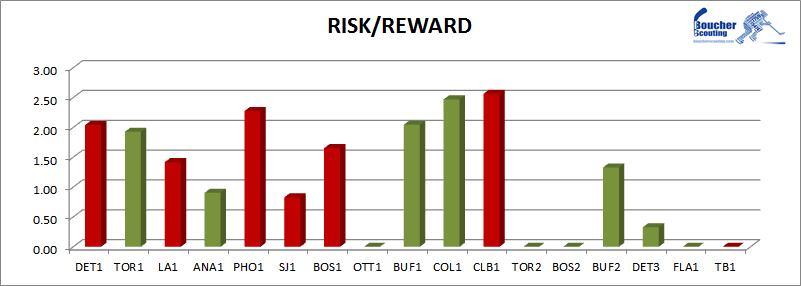The average ratio for Habs DEFENSEMEN prior to the Olympics was 2.84; since the games it's been 2.76.  Tinordi's average ratio prior to the Olympics was 1.93; since the games it's been 2.19.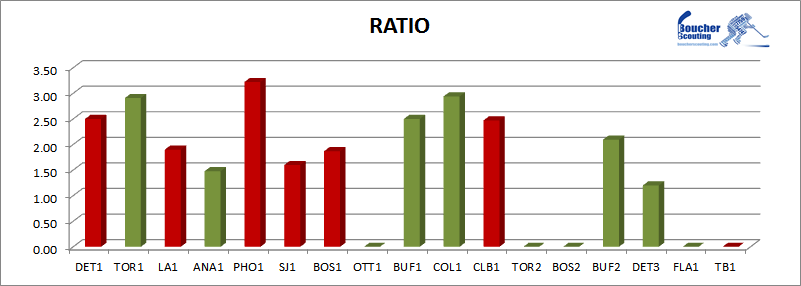JOSH GORGES
The average risk/reward rating for Habs DEFENSEMEN prior to the Olympics was 2.11; since the games it's been 2.00. Gorges' average risk/reward rating prior to the Olympics was 2.07; since the games it's been 1.68. That said, Gorges was playing with a broken hand for many of those games.
Gorges' injury made it difficult for him to produce an above-average rating.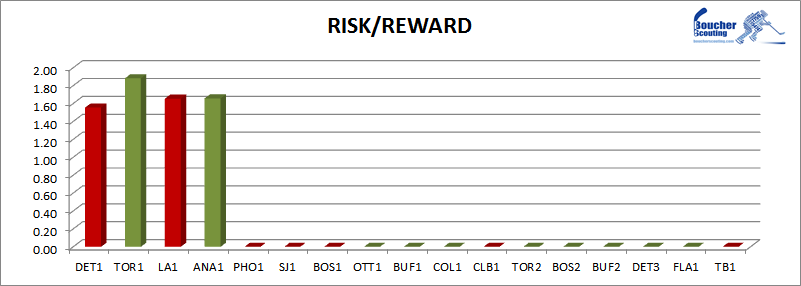The average ratio for Habs DEFENSEMEN prior to the Olympics was 2.84; since the games it's been 2.76.  Gorges' average ratio prior to the Olympics was 3.07; since the games it's been 2.62.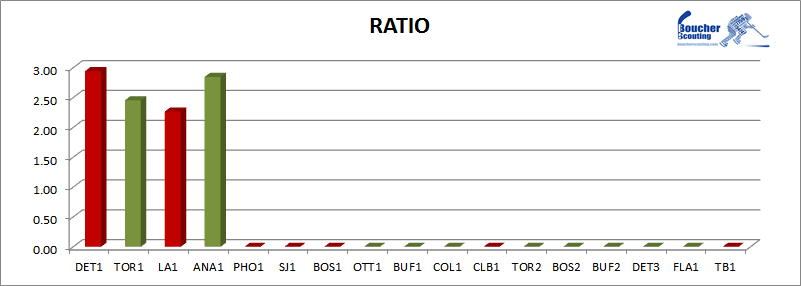ALEX GALCHENYUK
The average risk/reward rating for Habs WINGERS  prior to the Olympics was 1.26; since the games it's been 1.38. Galchenyuk's average risk/reward rating prior to the Olympics was 1.17; since the games it's been 1.30.
Since the Olympics, Galchenyuk has produced an above-average rating 7 times.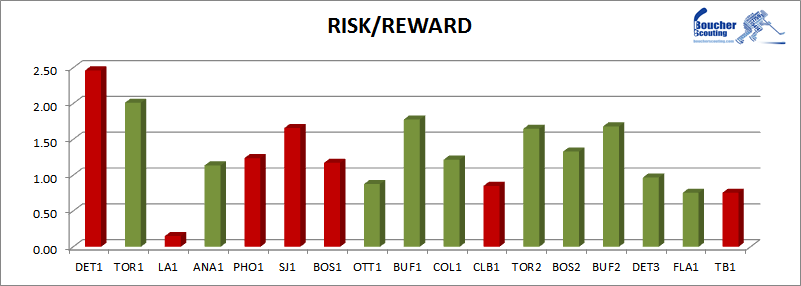The average ratio for Habs WINGERS prior to the Olympics was 1.88; since the games it's been 2.02.  Galchenyuk's average ratio prior to the Olympics was 1.86; since the games it's been 1.95.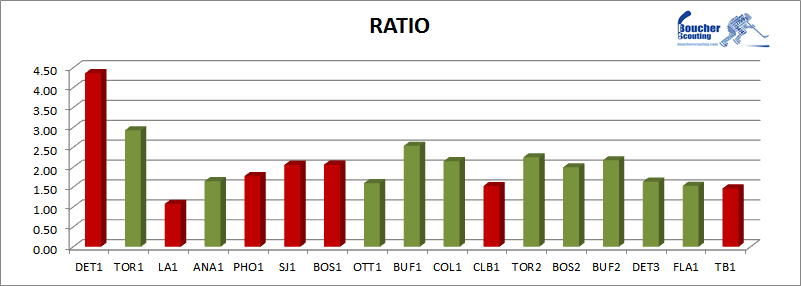TRAVIS MOEN
The average risk/reward rating for Habs WINGERS  prior to the Olympics was 1.26; since the games it's been 1.38. Moen's average risk/reward rating prior to the Olympics was 1.11; since the games it's been 1.33.
Since the Olympics, Moen has produced an above-average rating 7 times, and a negative-rating twice.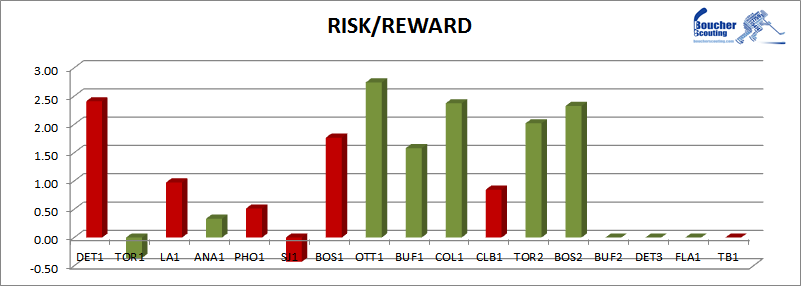The average ratio for Habs WINGERS prior to the Olympics was 1.88; since the games it's been 2.02.  Moen's average ratio prior to the Olympics was 1.85; since the games it's been 2.11.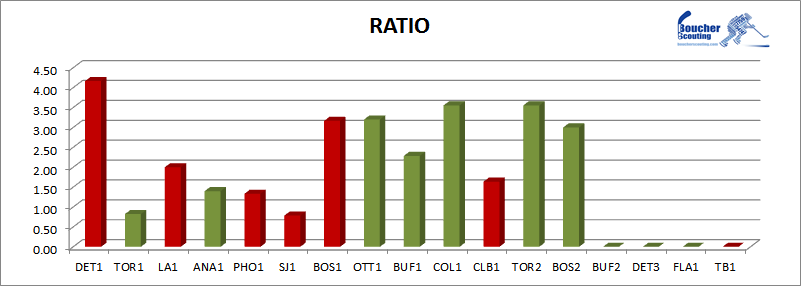MIKE WEAVER
The average risk/reward rating for Habs DEFENSEMEN prior to the Olympics was 2.11; since the games it's been 2.00. Since his arrival, Weaver's risk/reward rating has been 2.03.
Weaver has produced a rating above the average for his position during 5 of 12 games. All five instances occurred over the last 8 games.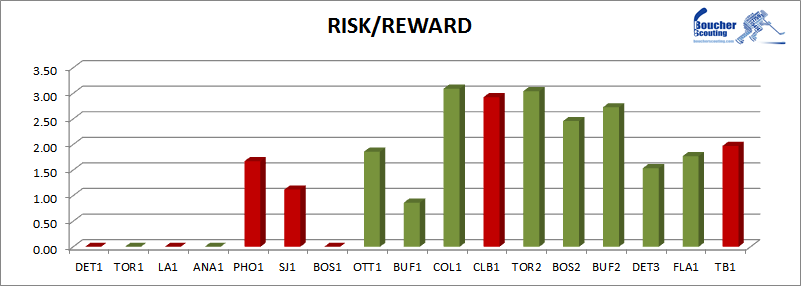The average ratio for Habs DEFENSEMEN prior to the Olympics was 2.84; since the games it's been 2.76.  Since his arrival, Weaver's ratio has been 3.05.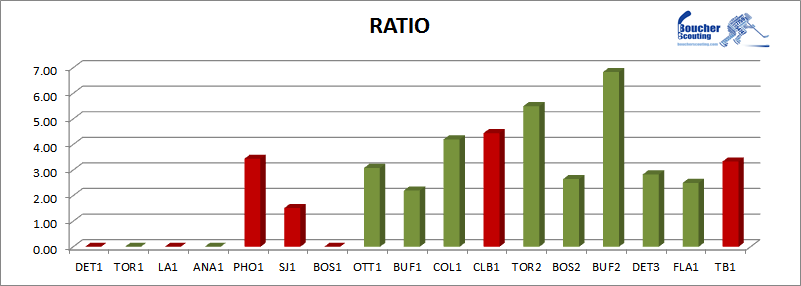DANIEL BRIERE
The average risk/reward rating for Habs WINGERS  prior to the Olympics was 1.26; since the games it's been 1.38. Briere's average risk/reward rating prior to the Olympics was 0.94; since the games it's been 0.95.
Since the Olympics, Briere has produced an above-average rating in 4 of 16 games.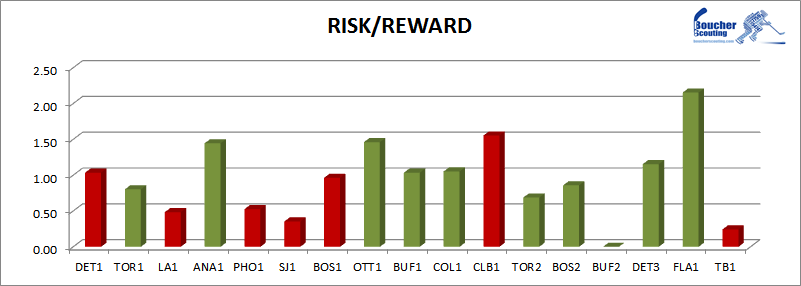The average ratio for Habs WINGERS prior to the Olympics was 1.88; since the games it's been 2.02.  Briere's average ratio prior to the Olympics was 1.71; since the games it's been 1.76.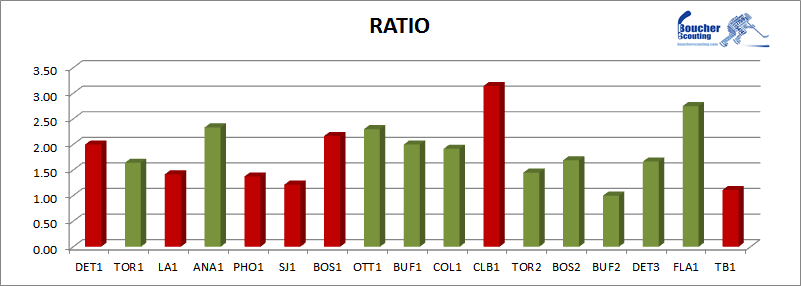MICHAEL BOURNIVAL
The average risk/reward rating for Habs WINGERS  prior to the Olympics was 1.26; since the games it's been 1.38. Bournival's average risk/reward rating prior to the Olympics was 1.60; since the games it's been 1.94.
Since his return from injury, Bournival has produced an above average rating in 4 of the 5 games he's played.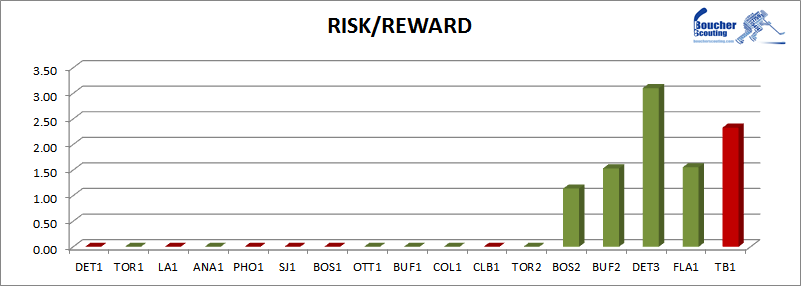The average ratio for Habs WINGERS prior to the Olympics was 1.88; since the games it's been 2.02.  Bournival's average ratio prior to the Olympics was 2.08; since the games it's been 2.42.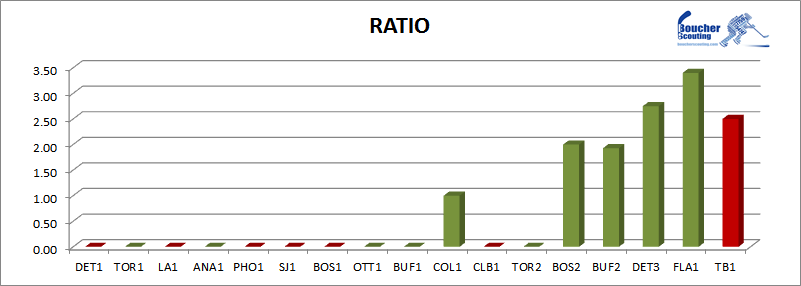DAVID DESHARNAIS
The average risk/reward rating for Habs CENTRES  prior to the Olympics was 1.57; since the games it's been 1.52. Desharnais' average risk/reward rating prior to the Olympics was 1.32; since the games it's been 1.40.
Since the Olympics, Desharnais has produced an above-average rating 6 times. Five of those instances have occurred over the last 9 games, but none during the most-recent 4 games.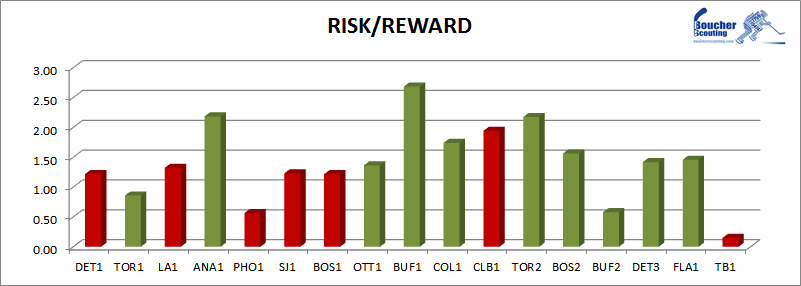The average ratio for Habs CENTRES prior to the Olympics was 2.20; since the games it's been 2.22.  Desharnias' average ratio prior to the Olympics was 2.06; since the games it's been 2.23.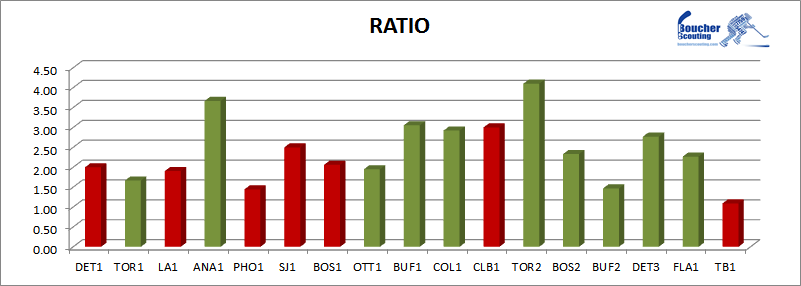RYAN WHITE
The average risk/reward rating for Habs CENTRES  prior to the Olympics was 1.57; since the games it's been 1.52. White' average risk/reward rating prior to the Olympics was 1.57; since the games it's been 1.52.
White has produced an above-average rating in 4 of the 11 games he's played since the break.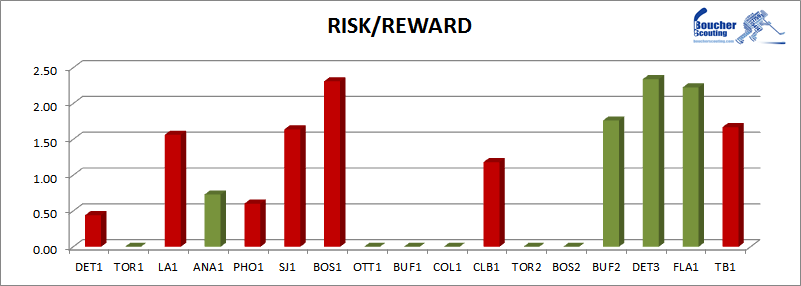The average ratio for Habs CENTRES prior to the Olympics was 2.20; since the games it's been 2.22.  White's average ratio prior to the Olympics was 2.42; since the games it's been 2.26.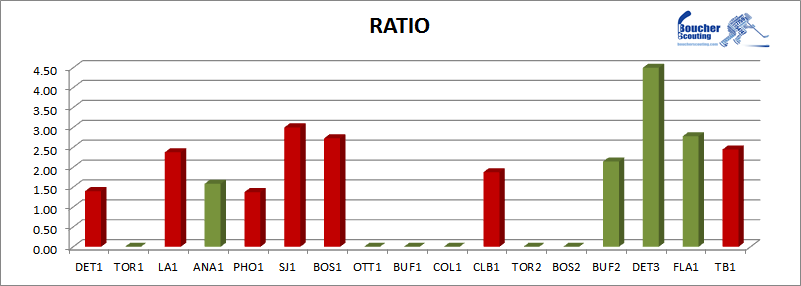FRANCIS BOUILLON
The average risk/reward rating for Habs DEFENSEMEN prior to the Olympics was 2.11; since the games it's been 2.00. Bouillon's average risk/reward rating prior to the Olympics was 1.79; since the games it's been 1.95.
Since his return to the lineup, Bouillon has produced an above-average rating in 5 of 10 games.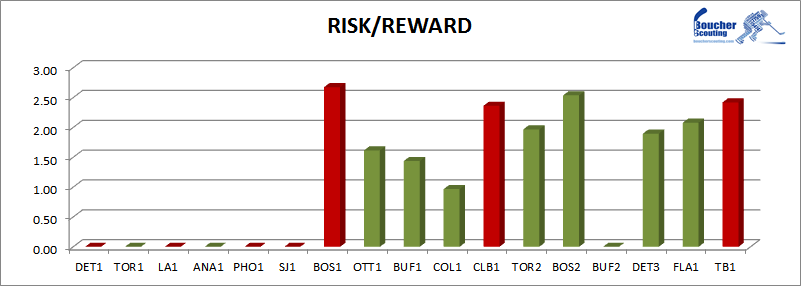The average ratio for Habs DEFENSEMEN prior to the Olympics was 2.84; since the games it's been 2.76.  Bouillon's average ratio prior to the Olympics was 2.85; since the games it's been 3.34. Bouillon's ratio has been above-average in each of the last 5 games he's played.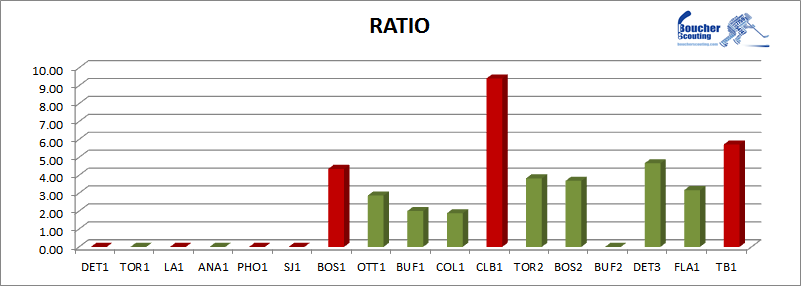MAX PACIORETTY
The average risk/reward rating for Habs WINGERS  prior to the Olympics was 1.26; since the games it's been 1.38. Pacioretty's average risk/reward rating prior to the Olympics was 1.50; since the games it's been 1.68.
Pacioretty's rating has been above-average in 10 games since the break.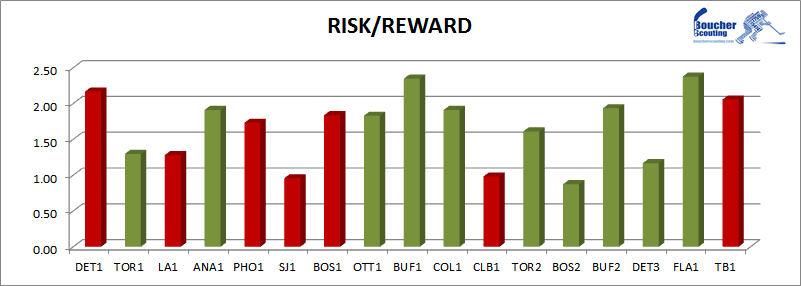The average ratio for Habs WINGERS prior to the Olympics was 1.88; since the games it's been 2.02.  Pacioretty's average ratio prior to the Olympics was 1.92; since the games it's been 2.06.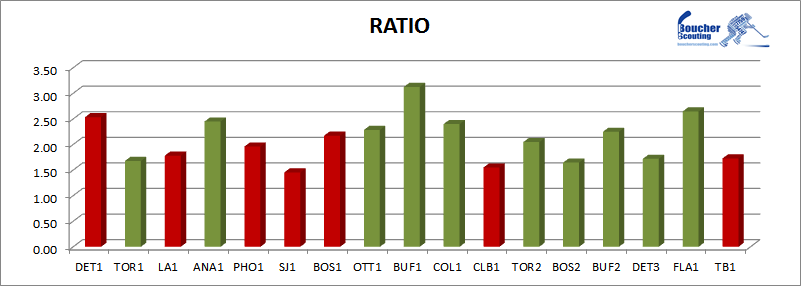ALEXEI EMELIN
The average risk/reward rating for Habs DEFENSEMEN prior to the Olympics was 2.11; since the games it's been 2.00. Emelin's average risk/reward rating prior to the Olympics was 1.92; since the games it's been 1.72.
Emelin's rating has been above-average in five of the 17 games indicated here.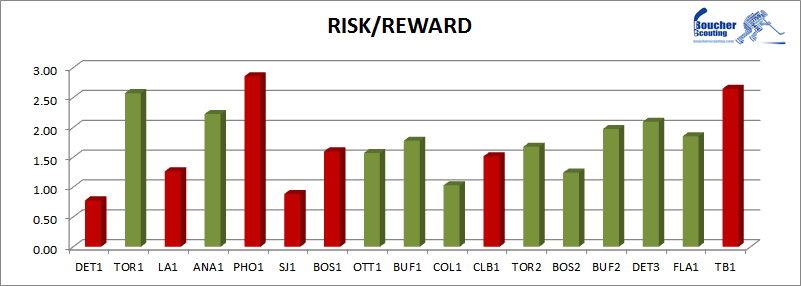The average ratio for Habs DEFENSEMEN prior to the Olympics was 2.84; since the games it's been 2.76.  Emelin's average ratio prior to the Olympics was 2.62; since the games it's been 2.41.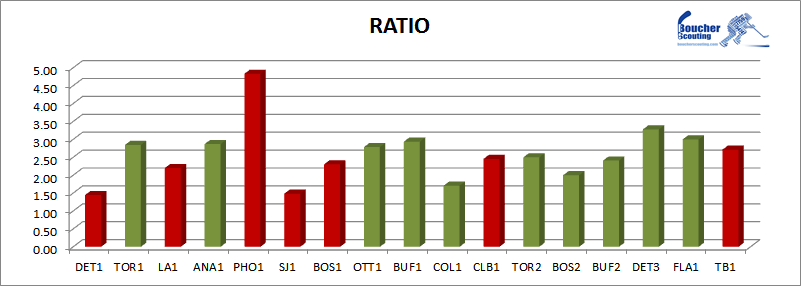PK SUBBAN
The average risk/reward rating for Habs DEFENSEMEN prior to the Olympics was 2.11; since the games it's been 2.00. Subban's average risk/reward rating prior to the Olympics was 2.58; since the games it's been 2.50.
Since the Olympics, Subban's rating has been above-average 13 times.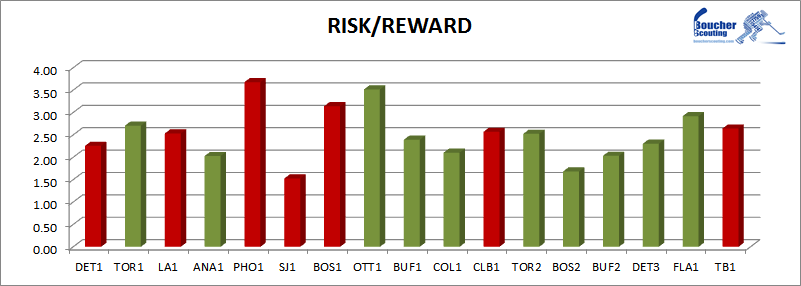The average ratio for Habs DEFENSEMEN prior to the Olympics was 2.84; since the games it's been 2.76.  Subban's average ratio prior to the Olympics was 3.14; since the games it's been 3.36.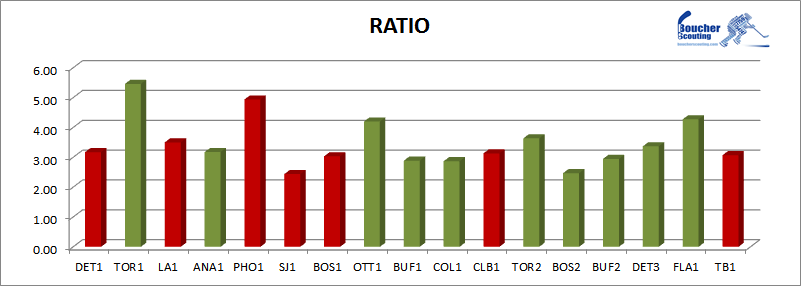ANDREI MARKOV
The average risk/reward rating for Habs DEFENSEMEN prior to the Olympics was 2.11; since the games it's been 2.00. Markov's average risk/reward rating prior to the Olympics was 2.26; since the games it's been 2.29.
Since the games, Markov's rating has been above the average among defensmen 15 times.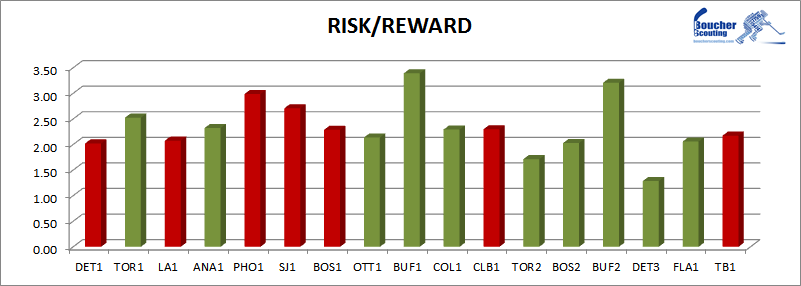The average ratio for Habs DEFENSEMEN prior to the Olympics was 2.84; since the games it's been 2.76.  Markov's average ratio prior to the Olympics was 2.91; since the games it's been 3.04.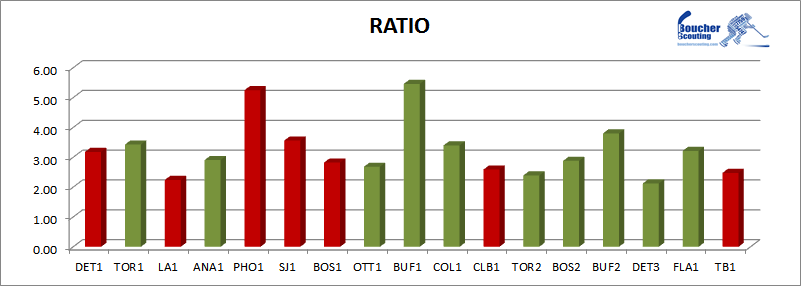LARS ELLER
The average risk/reward rating for Habs CENTRES  prior to the Olympics was 1.57; since the games it's been 1.52. Eller' average risk/reward rating prior to the Olympics was 1.93; since the games it's been 1.67.
Since the Olympics, Eller's rating has been above-average 8 times.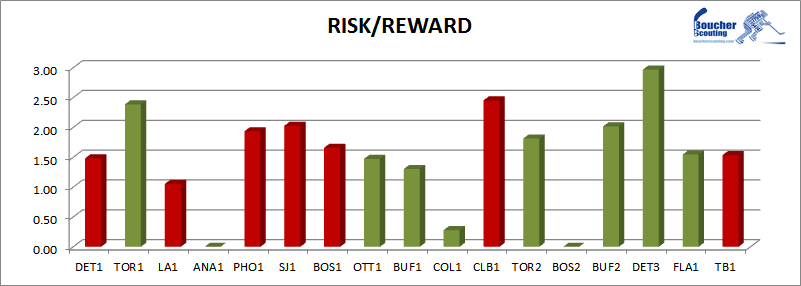The average ratio for Habs CENTRES prior to the Olympics was 2.20; since the games it's been 2.22.  Eller's average ratio prior to the Olympics was 2.31; since the games it's been 2.09. Eller's ratio has been above-average only 6 times since the Olympics.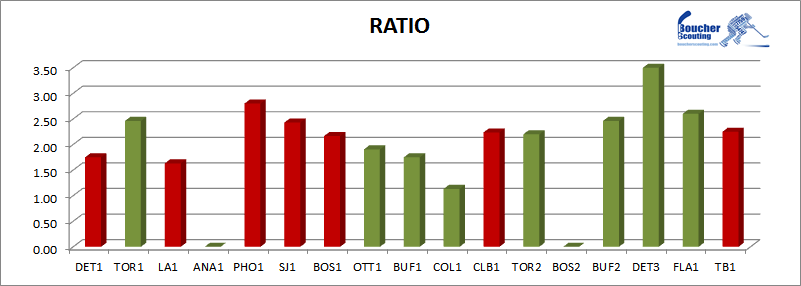These reports can be produced for any player at any level. All that's required is video. Feel free to contact me by e-mail with any questions, comments, or inquiries about the services I provide.
[email protected]

See more